Courses

# Differential Calculus MCQ Level - 1

## 10 Questions MCQ Test Topic wise Tests for IIT JAM Physics | Differential Calculus MCQ Level - 1

Description
This mock test of Differential Calculus MCQ Level - 1 for IIT JAM helps you for every IIT JAM entrance exam. This contains 10 Multiple Choice Questions for IIT JAM Differential Calculus MCQ Level - 1 (mcq) to study with solutions a complete question bank. The solved questions answers in this Differential Calculus MCQ Level - 1 quiz give you a good mix of easy questions and tough questions. IIT JAM students definitely take this Differential Calculus MCQ Level - 1 exercise for a better result in the exam. You can find other Differential Calculus MCQ Level - 1 extra questions, long questions & short questions for IIT JAM on EduRev as well by searching above.
QUESTION: 1

### The functIon u(x, y) = x2 + xy + 3x + 2y + 5 has a point of :

Solution:

Let z = x2 + xy + 3x + 2y + 5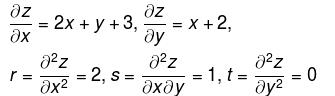For max. or min of z, we must have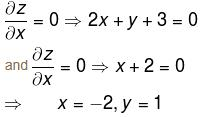at (–2, 1)
rt – s2 = –1 < 0
∴  neither max. nor min.
The correct answer is: neither max nor min

QUESTION: 2

###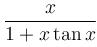is maximum at x= :

Solution: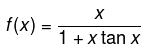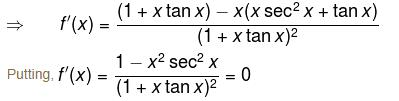We get, x = ±cos x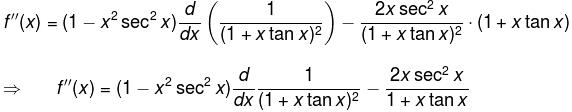∴ f(x) has maximum where x = cos x.

The correct answer is: cos x

QUESTION: 3

### The points on  z2 = xy + 1  nearest to the origin are :

Solution:

Let l be the distance from (0, 0, 0) to (x, y, z) on the surface
z2 = xy + 1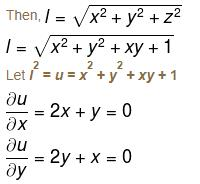Solving, we get
x = 0, y = 0
r = 2, s = 1, t = 2
rt – s2 = 3 > 0 and r > 0
Thus, minimum at (0, 0)
When, x = 0, y = 0, z = ±1
So, the points are (0, 0, 1) and (0, 0, –1)

The correct answer is: (0, 0, 1) and (0, 0, –1)

QUESTION: 4

The values of x1 and x2 with x1 < x2  such that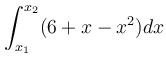has the largest value are :

Solution: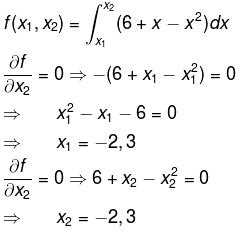Since, x1 < x2
So, x1 = –2  &  x2 = 3

The correct answer is: –2, 3

QUESTION: 5

The sum of two number is k. The minima value of the sum of their squares is :

Solution:

Let the two numbers be x and y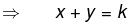and let z be the sum of squares.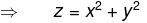x2 + (k – x)2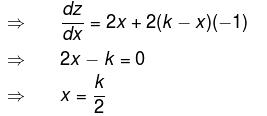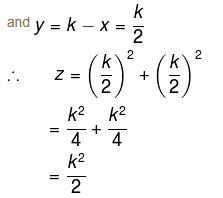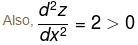Hence, z is minimum at x = k/2 and its minimum value is k2/2.

QUESTION: 6

The maximum value of f(x) = (1/x)x is

Solution: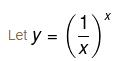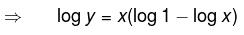= –x log x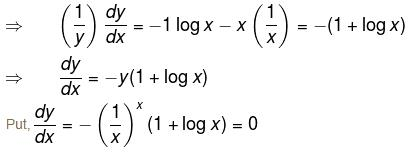i.e., 1 + log x = 0
i.e., log x = –1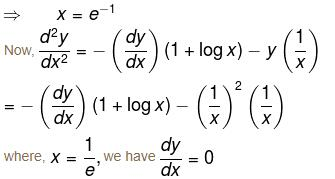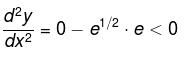Hence, y is maximum where x = 1/e and maximum value is e1/e.

QUESTION: 7

For the function x2 + y2,  (0, 0) is a point of :

Solution:

Let f(x, y) = x2 + y2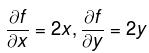For stationary points,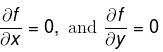⇒ (0, 0) is the only stationary point
r = fxx = 2, t = fyy = 2, s = fxy = 0
rt – s2 = 4 – 0 = 4 > 0 and r > 0
Thus, (0, 0) is a point of minima.

QUESTION: 8

If  dy/dx = (x - a)2n (x - b)2p + 1,  where n and p are positive integers, then at x = a, x = b, f(x) has respectively :

Solution:

For stationary points, put dy/dx = 0
i.e. (x – a)2n(x – b)2p+1 = 0
i.e. x = ab
Nature at x = a : Since 2n is even, dy/dx does not change its sign while x passes through a.

∴  Neither maximum nor minima at x = a.

Nature at x = b : when x is slightly less than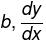is negative as 2p + 1 is odd.

Also, when x is slightly more than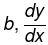is positive. Thus, y is minimum at x = b.

The correct answer is: neither maxima nor minima, minima

QUESTION: 9

The maximum value of (x – 1)(x – 2)(x – 3) is :

Solution:

f(y) = (x – 1)(x – 2)(x – 3)
= x3 – 6x2 + 11x – 6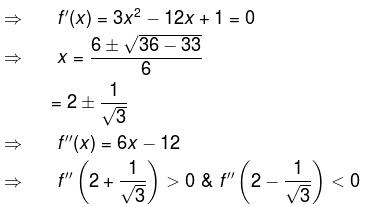Hence, f(x) has minima at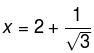and f(x) has maxima at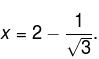∴  the maximum value of f(x) is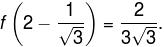The correct answer is: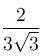QUESTION: 10

The saddle point of the function z = x2y – y2x – x + y is :

Solution:

For max. or min.,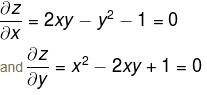Solving, we get
x = 1, y = 1
x = –1, y = –1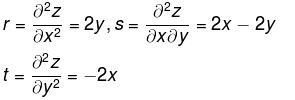at (1, 1)     r = 2, s = 0, t = –2
rt – s2 = –4 < 0

∴ neither max nor min at (1, 1)
at (–1, –1)  r = –2, s = 0, t = 2
rt – s2 = –4 < 0
Thus, (–1, –1) is also a saddle point.

The correct answer is: both (1, 1) and (–1, –1)